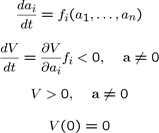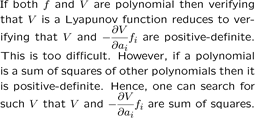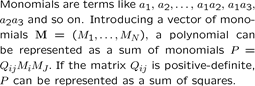# Polynomial sum of squares in fluid mechanics

## Non-linear stability analysis of fluid flows using polynomial sum of squares

#### Lyapunov function#### Polynomial Lyapunov functionStability of a dynamical system with respect to finite perturbations can be established by finding a Lyapunov function. However, there is no general systematic method for constructing Lyapunov functions -- the discovery of such a function is dependent on the ingenuity and creativity of the investigator. Fortunately, in 2000 a breakthrough in control theory, made in a PhD thesis by P.Parrilo, has provided a constructive method for generating Lyapunov functions for systems whose dynamics can be described by polynomial functions. This method is based on sum-of-squares (SOS) optimization, which reduces the problem of finding a Lyapunov function for a polynomial system to one of constructing a polynomial function that satisfies a selection of algebraic conditions. Using a number of key results from semialgebraic geometry, in particular the Positivestellensatz theorem, the resulting problem can be reformulated as an optimization problem in the form of a Semi-Definite Programme (SDP). This is particularly promising since SDP optimization problems are convex and tractable (e.g. solvable in a number of operations that is a polynomial function of the problem size). A variety of well-supported software codes for solving such problems are freely available.

#### Polynomial being a SOS is equivalent to a matrix being positive-definiteIt is well known that the Galerkin approximation of the incompressible Navier-Stokes equations leads to an ODE with quadratic non-linearity. The SOS approach can be applied to such a system since the dynamics are represented by polynomials. SOS was applied to a hydrodynamic-type ODE system of the 9th order. A method of exploiting in the SOS approach the energy-invariance property of the bilinear component of the systems of hydrodynamic type was developed. This allowed the construction of a Lyapunov function for the value of the Reynolds number about seven times larger than the largest value for which non-linear stability of this system can be proven by the standard energy stability approach. This demonstrates the strong potential of applying SOS in fluid dynamics.

Accurate approximation of a fluid flow requires considering systems with a large number of Galerkin modes. However, application of conventional SOS methods to very large systems is problematic, since the SDP problems to be solved in such cases are prohibitively large. A method of reducing the order of the system in SOS studies, based on representing the higher modes with their energy only, has been developed. The method is guaranteed always to give results at least as good as the standard energy stability analysis. Moreover, it has also been proven mathematically that if the flow remains globally stable for the Reynolds number range beyond that given by the energy stability analysis, then there exists a polynomial Lyapunov function giving a better stability bound than the energy stability theory.